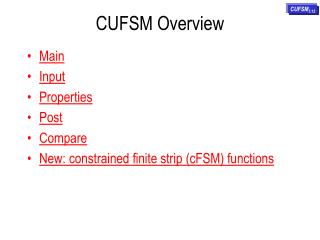DownloadDownload PresentationCUFSM Overview

# CUFSM Overview

Télécharger la présentation## CUFSM Overview

- - - - - - - - - - - - - - - - - - - - - - - - - - - E N D - - - - - - - - - - - - - - - - - - - - - - - - - - -
##### Presentation Transcript

1. CUFSM Overview CUFSM3.12 • Main • Input • Properties • Post • Compare • New: constrained finite strip (cFSM) functions

2. 1 2 4 3 Load and Save files as desired. To compare more than one analysis, save several different files after performing analysis and load them into the Comparison post-processor (start this by pressing Post). Print, sends the current screen to the default printer. Copy sends the current screen to the clipboard in bitmap format. Reset starts the program over and clears all entered data. Exit, leave CUFSM Z: zoom in and zoom out R: rotate 1. Enter the Geometry2. Apply the desired load/stress distribution3. Analyze the member4. Post-process and recover modes and critical loads

3. Define as many materials as you like here. • This is the input page. • An example is built-in to CUFSM (see Tutorial 1) and that example always comes up when CUFSM is started. • Tutorial 1 demonstrates the basic functionality of this page and many of the different plotting options. • Tutorial 2 shows how to enter a member from scratch and how the Double Elem. button may be used to improve your model. • Tutorial 3 shows how to use the C/Z template to input a member. Directly enter, or cut and paste in,the geometry of your member here. Define the elements here. Each element has an individual thickness and material that you select. Define the half-wavelengths that your member will be analyzed at here. Define constrained finite strip analysis parameters, and which modes to analyze You can model any external springs that are attached to your member here. You can model any equation constraints for your mode here.

4. Simple member properties are calculated and given above. These are used below to determine stress distributions on the member. Generate bimonent based on torqre (T) you input and L, x. The boudary condition is simply-simply supported beam with length L and the location of torque is at x along the length. An example will be given in next slide. Tutorials 2 and 3 show how to use this section effectively for simple Cee and Zee members. By defining a maximum, or yield stress, loads (P) and moments (M) may be determined. Any of these P or M can be used to generate a reference stress distribution on the member you create in the Input page.

5. By setting torque T=50, Length L=100, and at x=50, the bimoment B is 2456.3212. Left is the distribution of warping stress for the default channel section. Check here and generate warping stress.

6. All plotting of the buckling mode shapes is controlled to the left. The key buttons are the arrows that control the half-wavelength. 2D and 3D plots of the mode shapes are available as well as a plot of the stress distribution. Values associated with the currently shown plot are given above the plot. Tutorials 1,2 and 3 show how to effectively interpret and manipulate the post-processing page. Full control over the buckling curve given below is available using the controls to the left. The numerical results for the first mode may be written to a text output file, if desired. The “red dot” on the plot shows where you are at on the plot, at all times.

7. The user has complete control over which buckling mode to view - select the type of plot, the half-wavelength, the mode, and which file you want to view! Multiple files are loaded for post-processing at the same time, see list to the left. You decide which loaded files should show in the buckling curves to the right.

8. To do modal classification, you must first turn cFSM on in “INPUT”, as shown in the figure below. See the tutorial on “advanced functions” for more on how to use cFSM. Modal classification: you can decide which norn to use, vector norm, strain energy norm and work norm. In this figure we use the strain energy norm. You can also see a supplemental participation plot.